# Solving Exponential Equations

in STEMGeeks2 years ago

Hi there. In this mathematics post, I cover solving exponential equations. This post will cover various cases through examples (without logarithms).Pixabay Image Source

## Topics

• Exponential Equations & Exponent Laws Basics Review
• Examples Of Solving Exponential Equations
• Practice Problems With Solutions

## Exponential Equations

An exponential equation is an equation that contains an unknown value that is an exponent. Algebra and exponent laws are used as math tools to solve such exponential equations.

Do be careful with a variable to an exponent such as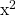and a number base to an unknown exponent such as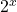. This post will cover solving unknown exponents.

Exponent Laws Basics Review

Given a common base `a` and different exponents we have: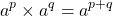Given a base `a` and negative exponent we have the reciprocal. Keep in mind there is no negative sign in this.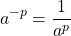For the power to a power case, take the product of the exponents.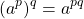Pixabay Image Source

## Examples Of Solving Exponential Equations

Example One

In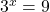, the value of `x` would be 2. Three squared is equal to 9.

Example Two

Solve for `x` in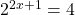. Rewrite the 4 from the right side as two-squared. Once the number bases are the same on both sides, compare the exponents and solve for `x`.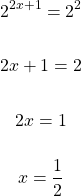Example Three

Solve for `x` in the equation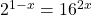.

Rewrite the 16 as 2 to the power of 4.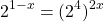Apply the power to a power exponent law on the right side. The new exponent with the number base of 2 would be the product of 4 and `2x` which is `8x`.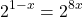As the number bases are the same, the variable `x` can be solved in the exponents.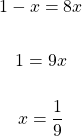Example Four

Solve for `x` in the equation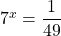.

Rewrite the number 49 as 7 squared on the denominator of the right side fraction.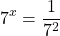The fraction on the right side can be rewritten as a number base to a negative exponent. This would be 7 to the power of negative 2. Comparing the exponents would give an answer of negative 2 for `x`.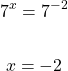Example Five

Solve for the value of `x` in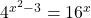.

Rewrite the 16 on the right side as four to the power of two with the exponent x on the outside.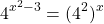Multiply the 2 and the x on the right side in the exponent.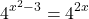As the bases are the same at 4, equate the exponents to each other and solve for `x`.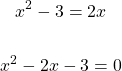This quadratic can be factored. Two numbers that multiply to negative 3 and add to negative 2 are -3 and 1.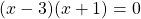The solutions here are 3 and -1.

Example Six

What is the value of `x` inRewrite the number 1000 as 10 to the power of 3.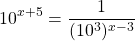The right side is a fraction that can be written as a base with a negative exponent.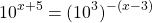Apply the power of a power exponent law on the right side. The distributive law is applied as well.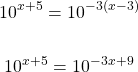There is now a common base of 10. The exponents from both sides can be equated to each other. After equating both sides to each other, the value of `x` can be solved for.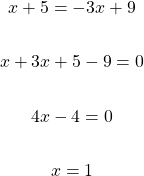Pixabay Image Source

## Practice Problems With Solutions

For the following exponential equations, solve for the value of `x`.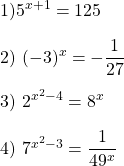Solutions

1. `x = 2`

2. `x = -3`

3. `x = -1 or x = 4`

4. `x = 1, x = -3`Pixabay Image Source